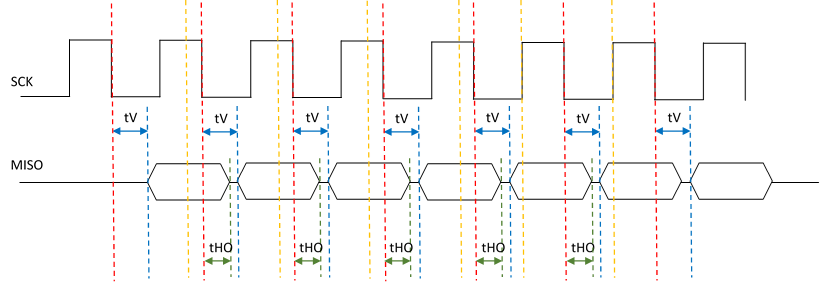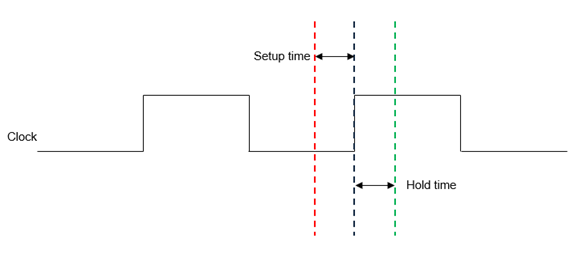cancel
Showing results for
Did you mean:

# Sampling Output Data from Cypress SPI NOR Flash Devices – KBA227433Employee

## Sampling Output Data from Cypress SPI NOR Flash Devices – KBA227433

Author:            Version: **

Figure 1.Question: How to sample output data from Cypress SPI NOR flash device, when tv (clock falling edge to output valid) time is maximum (tvmax)?

Please refer to Figure 1 to understand about how the tV parameter is defined from the flash point of view. The flash device will give data out during a read operation tV time after the clock falling edge and it will be available on the SPI bus until tHO time after the next clock falling edge. The data valid window for the MCU to sample the data correctly will be as follows:

Data valid window = Clock period (tPSCK) – tV(max) +tHO

The MCU should decide when to sample the data while reading from flash based on the data valid window. Therefore, the maximum frequency with which a read operation can be performed depends upon the  data valid window, the data setup time, and the data hold time requirements of the MCU.

Input setup time: The amount of time the data at the flash controller data input must be stable before an active edge of the clock.

Input Hold Time: The amount of time the data at the flash controller data input must be stable after an active edge of the clock.

Figure 2.See the following examples (S25FL256S😞

Let us assume the following:

• Load capacitance (CL) = 30 pF
• Operating voltage range (VCC) = 2.7 to 3.6 V
• tV parameter = 8 ns
• Operating frequency (FSCK) = 100 MH(tPSCK = 10 ns)

Based on this formula, the data valid window can be calculated as follows:

Data valid window = tPSCK – tV(max) + tHO = 10 – 8 + 2 = 4 ns.

Let us consider the setup time and hold time requirements of the MCU as follows:

Example 1:

• Input setup time of MCU = 2 ns
• Input hold time of MCU = 2 ns[YQ1] [SK2] [YQ3]

Minimum data valid window required = Setup time + Hold time = 4 ns

Therefore, it is possible to sample the data along with the falling edge of the same SPI clock by the MCU. See the red line in Figure 2.

Example 2:

• Input setup time of MCU = 5 ns
• Input hold time of MCU = 2 ns

Minimum data valid window required = Setup time + Hold time = 7 ns

Because the data valid window available is only 4 ns, the MCU will not be able to sample the output data correctly in this case. The SPI clock frequency should be reduced to make the data valid window wider such that the setup time and hold time of the MCU are met.

Do the following to make the data valid window wide if the setup time and hold time requirements of the MCU are not met.

1. Reduce CL to reduce the tV value.
2. Reduce the SPI clock frequency.
Version history
Revision #:
1 of 1
Last update:
‎May 31, 2019 12:17 AM
Updated by:
Contributors